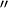# Arcsecond facts for kids

Kids Encyclopedia Facts

An arcsecond (second of arc), represented by the symbol$''$, is a unit of angle used in mathematics and astronomy. An arcsecond is 1/60th of an arcminute (minute of arc), which in turn is 1/60th of a degree. Therefore, an arcsecond is equal to 1/3600th of a degree, meaning that there are 360 × 60 × 60 = 1,296,000 arcseconds in a full circle.

## Images for kidsArcsecond Facts for Kids. Kiddle Encyclopedia.